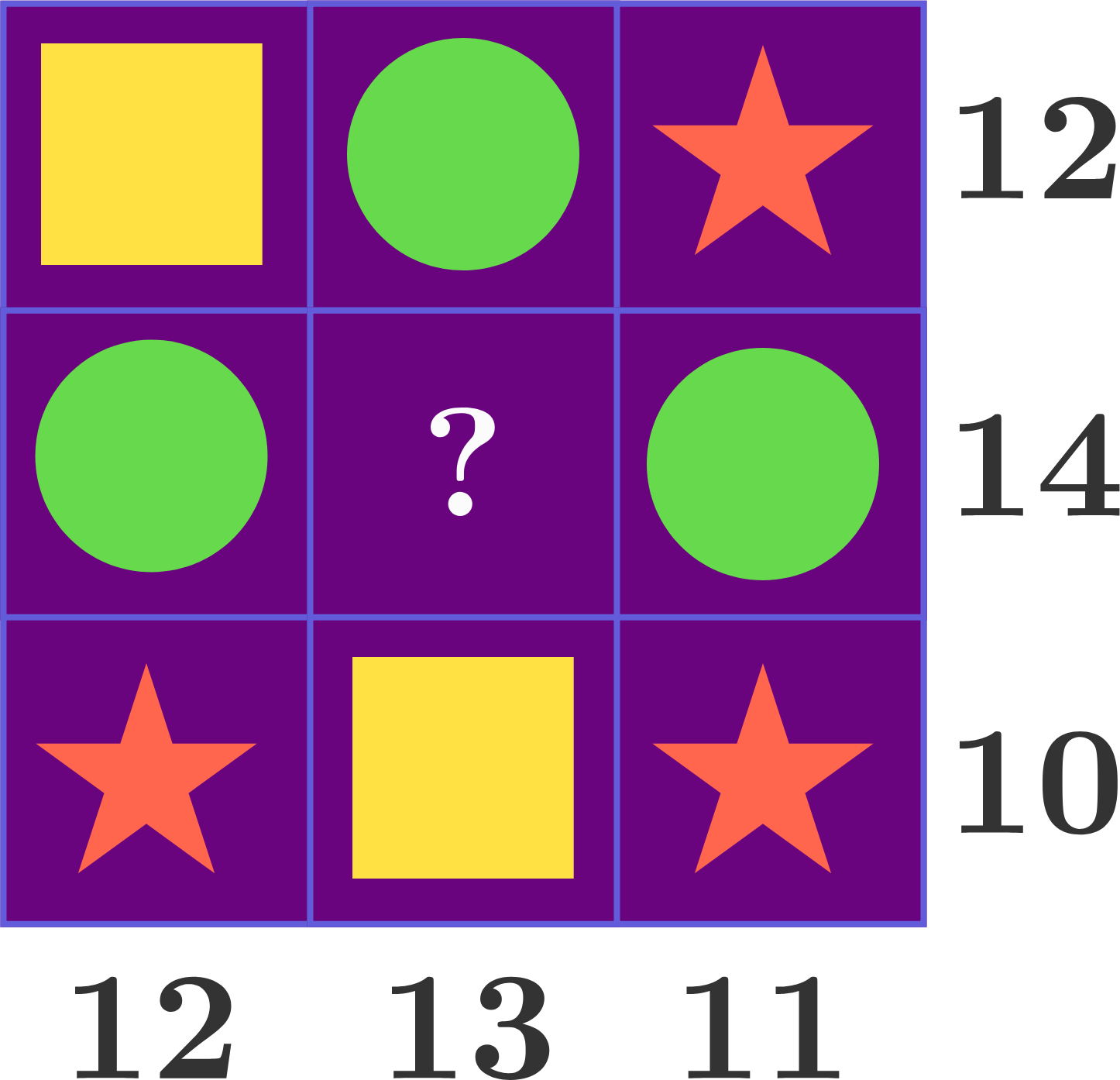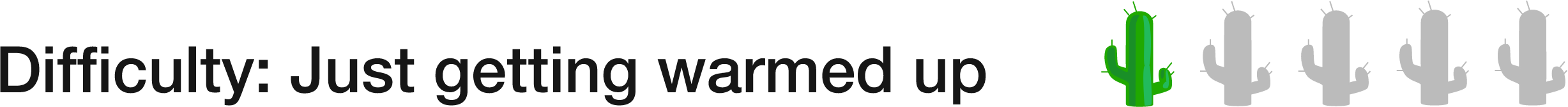# 5 of 100: A Sum of Shapes

Algebra Level 1Each shape in this puzzle represents a numerical value. The number next to each row or column represents the sum of all of the values in that row or column. Can the center of the grid be filled in with one of the three shapes? If so, which one?While it is possible to solve for the value of each symbol, there is a quick and clever way to solve this problem without finding the specific value of any of the shapes.

×